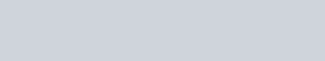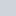# DC Water Heating

## DC Water Heating Using Low Voltage ElementsWe can use the sun’s solar energy directly for domestic hot water heating systems by using solar thermal panels and evacuated tubes. But we can also use the DC (direct current) power generated from photovoltaic panels or turbine generators to power a DC Water Heating Element without the use of mains electricity.

When an electrical current flows through a resistive element, heat is produced and the element becomes red hot. Thus the I2R heating effect of an electrical current passing through a high resistance element is the basic principle for all heating elements. Whether it is a wire heating element, electric kettle, or electric iron, etc.

For most domestic hot water systems with storage tanks, we would typically use a conventional tubular rod type AC (alternating current) resistive heating element powered by the utility company to heat the water. The amount of heating power, in “watts” that can be produced by a resistive element depends on the mains voltage supplied to it, as well as its static cold resistance. That said, there are a whole range of 120-volt and 240-volt electric heating elements available to choose from rated at between 100 to 5,000 watts.

Also, resistive heating elements can be considered as being 100% efficient, as all of the electrical input energy is converted into a useful output, in this case heat. The amount of electrical energy converted or consumed into thermal energy is measured in Watts.DC Water Heating Element

For DC circuits, the power in watts is equal to the product of volts and amperes. That is V x I. However, for AC circuits, the power in watts is equal to the product of the volts times amperes at that instant in time.

The AC voltage used in a typical household is expressed in terms of its effective value. This effective voltage value is more commonly known as the “Root Mean Squared”, or RMS voltage value. For example 120 volts rms (120 Vrms), or 240 volts rms (240 Vrms).

The RMS value commonly given of an AC voltage, produces the equivalent amount of heating effect when applied to a resistive heating element as would a direct current (DC) voltage of the same voltage value. That is: 120 Vrms = 120 Vdc.

Ohm’s Law tells us that if the RMS voltage, in volts (V) of the supply, and the resistance in ohms (Ω) is known. Then the power (P) dissipated by a heating element, in watts (W), can be given by the formula of: P = V2/R watts. Likewise, if we know the current (I) that the water heating element draws in amperes (A) along with the voltage. Then the power (P) dissipated by a heating element, in watts (W), would be given by the formula of: P = V*I [watts].

## DC Water Heating Example No1

So for example. If a 2400W heating element is connected to a 240 volt supply, what will be the current taken from the supply, and the static cold resistance of the element.

1.  current taken from the supply:2.  heating element resistance:Then we can see that for our example element, its cold resistance is 24 Ohm’s, (no matter what voltage is applied) and it will consume 10 Amperes of current at 240VAC. Heating elements designed for 120 or 240 volt power supplies commonly have a much higher resistance. By using the voltage and the elements fixed resistance, the electric power dissipated by it can be calculated.

For example, suppose we use the same 240 volt, 2400 watt heating element from example No1 above on a 120 volt AC supply, what will be the power output of the element.

### Power from a 120 Volt SupplySo by using our 240V rated heating element on a 120V supply would reduce the power output by 75% to 600 watts. We would expect to see this as every time the voltage is cut in half, the wattage drops by 75% due to the squaring of the voltage within the power formula. In other words, the 240V elements heating effect is reduced to 1/4 of its original output when connected to a 120V supply. But would the same heating element heat up water using DC electricity from a turbine generator (wind or water) or solar panel.

## DC Water Heating Elements

Many people use solar energy and thermal collector panels for preheating their domestic hot water. But we can also use the DC output from photovoltaic panels or a pv array to heat water, we just have to think a little differently.

Heating water directly using a DC water heating element together with either a wind turbine generator or photovoltaic panel (with or without a battery bank) is commonly used in diy solar power systems and off-grid applications where there is no grid connection or any mains utility power. DC water heating elements along with a pv array can even provide heated water under low irradiation or bad weather conditions, as the only limit to how hot the water becomes depends on how much energy a heating element can deliver into it.

AC water heating elements will work using DC supplies. However, as we have seen, when the supply voltage is reduced on the same element, the heating effect of the element is also reduced. For example, let’s assume we want to use our 240 volt, 2400 watt element from above on a 24 volt DC supply. What will be the wattage generated by the heating element.

### DC Water Heating WattageAs calculated previously, the Ohmic rating of our element is fixed at 24 Ohms. But when connected to a 24 volt DC supply, the same heating element produces only 24 watts of power and therefore does not get as hot. That is, its heating effect is reduced to 1/10 of the original output when connected to a 240 volt supply. Then clearly, we need a DC water heating element that is rated for the low voltage supply as no matter which formula we use, only 24 Watts of power is dissipated as heat.

Low voltage DC water heating elements are readily available for off-grid applications such as the popular DERNORD 700W 12V DC Water Heater Element from Amazon that simply threads into your existing water heater tank.

These low voltage DC water heating elements can be powered using solar, wind, or battery, they can be powered directly from a single solar panel or pv array to heat up water with DC electricity. They can also be used as a dump load for a wind turbine. Clearly, the connected solar panel or turbine must have a similar wattage to the element.

DC immersion elements come in various voltages ranging from 12v to 48v, but you need to buy an element that is rated for your system voltage in order to sufficiently preheat your domestic hot water. So using the DERNORD 700W 12V DC Water Heater Element as our example. What will be its static cold resistance and current drawn from the 12 volt DC supply.

Remember that Ohm’s laws says: Volts squared ÷ Watts = Ohms, and Voltage ÷ Resistance = Current

1.  700 Watt heating element resistance:2.  Current taken from the supply:There are two things to note here. 1. The resistance of the heating element is much, much lower at only 0.21Ω’s (0.206Ω’s rounded off), 2. the current taken from the supply is much higher, at 58 Amperes. If we replaced it with a higher voltage 48VDC, 700W water heater element, then its values would be: 3.3Ω’s and 14.5 Amperes respectively, a much lower current value.

Then we can see that more current must be supplied by a panel or turbine with a lower voltage in order to provide the same amount of electric power, 700 watts in this example.

But we cannot just increase the voltage applied to our 12VDC element in order to reduce the current (and therefore cable size). What would happen when 48VDC is connected to the 12 volt 700W rated element which as a cold resistance of 0.21Ω’s. Well let’s use Ohm’s law again to find out.

P = V2 ÷ R = (48×48) ÷ 0.21 = 10,972 watts … the element will burn out immediately!

In other words, 10,972 watts (or 15 times the rating) running through a 700 watt element will cause it to burn out immediately. Then its important to understand that for a DC heating element its Ohmic value will vary by wattage and voltage rating. Fortunately, manufacturers commonly print the wattage and voltage rating on the side of each element for easy reference.

Using two or more heating elements in series is another option to use lower voltage elements on a higher voltage supply, since the voltage applied to a series combination of elements results in a portion of the voltage appearing across each of the elements. Thus two 12VDC heating elements could be used on a 24VDC supply. The electric current value will be the supply voltage divided by the sum of the element resistances.

## Tutorial Summary

We have seen here that DC water heating elements can be used to heat (or preheat) domestic hot water from a pv panel or array, or as a diversion load heating element for the over production from a wind (or hydro) turbine. However, it must be realised that selected element must be used with the designed voltage supply, as less supply voltage will reduced it power output, meaning that The heating element does not get as hot. While too much supply voltage means that the heating element will get too hot and will burn out.

There are a variety of low volt elements available online from 12VDC to 48VDC with power ratings from 100 to 1,000 watts. However, if you use a heating element at a lower voltage than its rated value, the wattage power output drops by 75% each time the supply voltage is halved.

While using low-voltage elements make sense for dissipating any excess solar electricity, thermal solar collectors and heating system dedicated to heating hot water using the sun’s energy are more cost effective. This is due in part to the higher efficiencies of solar thermal collectors and the lower cost per square foot of collector/panel surface area.

Related Tags:

•Pico Solar Systems
•Battery Charge Controller
•Parallel Connected Solar Panels
•Series Connected Solar Panels
•Measuring the Power of a Solar Panel
•Solar Irradiance
•Understanding Batteries
•DIY Solar Power System

We hope this DC Water Heating Tutorial was useful and informative for you. Are you ready to share your thoughts
and experience with us and many others. Your comments are always welcome, just post them in the section below.

P.S. Don't forget to like, rate, and share this Alternative Energy Tutorials post. Thank you for using our website.

• Shane McGovern says:

I have an old HWS with a 240 Volt 3600 Watt therefore a 16 Ohm resistance.
Can I use say 6×36 Volt solar panels (5.4 Amps each) in series to give 216 volts 5.4 Amps.
That would mean my output power to heat the water would be 216×216/16 giving 2916 Watts.
Would that be OK.

• Ok, some basic maths. Data given about resistive heating element: 240V, 3600W. Then I = 15 Amperes (at 240V) and R = 16 Ohms.

PV panel data. Vmp = 36V, Imp = 5.4A, therefore P = V x I = 36 x 5.4 = 200 watts approx. per panel or 200 x 6 = 1200W total.

PV voltage of series string = 1200/5.4 (amperes are common) = 222 volts DC.

If PV current = 5.4 Amperes, power supplied to resistive heating element equals:

P = I2x R = 5.42 x 16 = 467 Watts. That is, 13% of heating capacity at full sun. Then you decide.

• Thomas Mann says:

I am looking to combine several technologies to go off grid